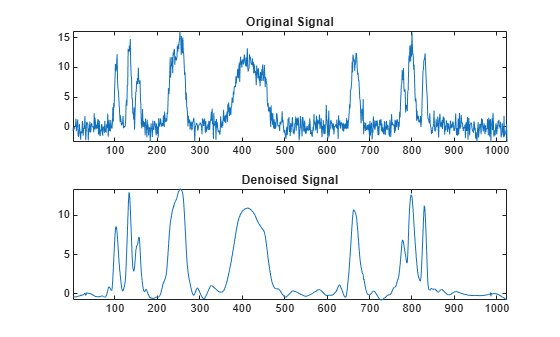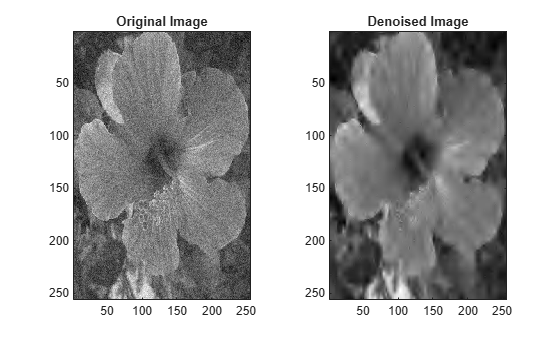# wbmpen

Penalized threshold for wavelet 1-D or 2-D denoising

## Syntax

``thr = wbmpen(c,l,sigma,alpha)``
``thr = wbmpen(c,l,sigma,alpha,ARG)``

## Description

example

````thr = wbmpen(c,l,sigma,alpha)` returns the global threshold `thr` for denoising. `c,l` is the wavelet decomposition structure of the signal or image to be denoised. `sigma` is the standard deviation of the zero mean Gaussian white noise in the denoising model (see `wnoisest` for more information). `alpha` is a tuning parameter for the penalty term.```
````thr = wbmpen(c,l,sigma,alpha,ARG)` computes the global threshold and plots three curves: `2×sigma^2×t×(alpha + log(n/t))``sum(c(k)^2,k≤t)``crit(t)` where `n` is the number of coefficients and crit(t) = -sum(c(k)^2,k≤t) + 2×sigma^2×t×(alpha + log(n/t)) For more information, see Penalized Criterion.```

## Examples

collapse all

```load noisbump x = noisbump;```

Perform a wavelet decomposition of the signal at level 5 using the `sym6` wavelet.

```wname = "sym6"; lev = 5; [c,l] = wavedec(x,lev,wname);```

Estimate the noise standard deviation from the detail coefficients at level 1, using `wnoisest`.

`sigma = wnoisest(c,l,1);`

Use `wbmpen` to obtain a global threshold for signal thresholding, using the tuning parameter.

```alpha = 2; thr = wbmpen(c,l,sigma,alpha)```
```thr = 2.7681 ```

Use `wdencmp` for denoising the signal using the threshold with soft thresholding and approximation kept.

```keepapp = 1; xd = wdencmp("gbl",c,l,wname,lev,thr,"s",keepapp);```

Plot the original and denoised signals.

```subplot(2,1,1) plot(x) axis tight title("Original Signal") subplot(2,1,2) plot(xd) axis tight title("Denoised Signal")``````load noiswom nbc = size(map,1);```

Perform a wavelet decomposition of the image at level 3 using the `coif2` wavelet.

```wname = "coif2"; lev = 3; [c,s] = wavedec2(X,lev,wname);```

Estimate the noise standard deviation from the detail coefficients at level 1.

```det1 = detcoef2("compact",c,s,1); sigma = median(abs(det1))/0.6745;```

Use `wbmpen` for selecting a global threshold for image denoising.

```alpha = 1.2; thr = wbmpen(c,s,sigma,alpha)```
```thr = 39.2910 ```

Use `wdencmp` for denoising the image using the threshold with soft thresholding and approximation kept.

```keepapp = 1; xd = wdencmp("gbl",c,s,wname,lev,thr,"s",keepapp);```

Plot the original and denoised images.

```colormap(pink(nbc)) subplot(1,2,1) image(wcodemat(X,nbc)) axis equal axis tight title("Original Image") subplot(1,2,2) image(wcodemat(xd,nbc)) axis equal axis tight title("Denoised Image")```## Input Arguments

collapse all

Wavelet decomposition structure of a signal or image, specified by the vectors `c` and `l`. The vector `c` contains the wavelet coefficients. The bookkeeping vector `l` contains the number of coefficients by level. For more information, see `wavedec` and `wavedec2`.

Data Types: `double`

Standard deviation of the zero mean Gaussian white noise in the denoising model, specified as a scalar. For more information, see `wnoisest` and Penalized Criterion.

Data Types: `double`

Tuning parameter for the penalty term, specified as a scalar greater than 1. The sparsity of the wavelet representation of the denoised signal or image grows with `alpha`. Typically, ```alpha = 2```. For more information, see Penalized Criterion.

Data Types: `double`

## Output Arguments

collapse all

Global threshold for denoising, returned as a scalar. `thr` is obtained by a wavelet coefficients selection rule using a penalization method provided by Birgé-Massart. For more information, see Penalized Criterion.

collapse all

### Penalized Criterion

The global threshold `thr` is obtained by a wavelet coefficients selection rule using a penalization method provided by Birgé-Massart.

The global threshold minimizes the penalized criterion given by the following:

Let `t`* be the minimizer of

```crit(t) = -sum(c(k)^2,k≤t) + 2×sigma^2×t×(alpha + log(n/t)) ```
where `c`(k) are the wavelet coefficients sorted in decreasing order of their absolute value and `n` is the number of coefficients; then `thr` = |`c`(t*)|

 Birgé, Lucien, and Pascal Massart. “From Model Selection to Adaptive Estimation.” In Festschrift for Lucien Le Cam, edited by David Pollard, Erik Torgersen, and Grace L. Yang, 55–87. New York, NY: Springer New York, 1997. https://doi.org/10.1007/978-1-4612-1880-7_4.